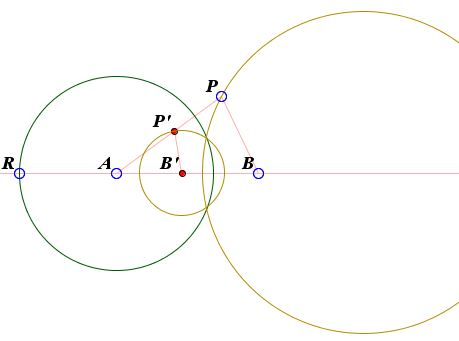Apollonian Circles Theorem

Given two points $A$ and $B$ and a number $r.$ What is the locus of points $P$ such that $AP/BP = r?$ The answer is a circle. The circle which is known as the Apollonian Circle. For every positive r there is a different one.

This problem has been treated elsewhere. Here we present a different solution based on the inversion transform. Along the way we show that the whole family of Apollonian circles can be inverted into a family of concentric circles.

SolutionApollonian Circles Theorem

For two fixed points $A$ and $B$ and a real $r\gt 0,$ the locus of points $P$ such that $AP/BP = r$ is a circle. The circle is known as the Apollonian Circle.Consider a circle of radius $R$ centered at $A.$ Let $t$ be the inversion in this circle. Set $t(B) = B'$ and $t(P) = P'.$ The calculations are simplified by taking $R = 1,$ but, in principle, any circle with center at $A$ will do. So, assume $R = 1.$

This means, in particular, that

(1)

$AP\cdot AP' = AB\cdot AB' = 1.$

Triangles $AB'P'$ and $APB,$ in which the sides satisfy (1), also share the angle at $A.$ They are, therefore, similar. It then follows that

(2)

$BP / B'P' = AB / AP'.$

From here,

(3)

$B'P' = BP\cdot AP' / AB.$

Combining (1) and (3) gives

(4)

\begin{align} B'P' &= BP / (AP\cdot AB)\\ &= 1/rAB. \end{align}

This tells us that $P'$ lies on a circle of radius $1/rAB$ centered at $B'.$ In other words, the inversive image of the locus of points $P$ is a circle centered at $B'.$ To repeat, the Apollonian circle defined by the point circles $A$ and $B$ and $r \gt 0$ is mapped by $t$ to a circle centered at $t(B).$ This is true for any Apollonian circle defined by $A$ and $B,$ so that the whole family of them is mapped on the family of concentric circles with center $t(B).$

In establishing Steiner's Porism we showed that any two non-intersecting circles can be inverted into a pair of concentric circles. The above strengthens this assertion with a more direct proof.

The Apollonian circles defined by two point circles are said to be coaxal. This is one of the three varieties of coaxal families.

References

1. D. A. Brannan et al, Geometry, Cambridge University Press, 2002Inversion - Introduction